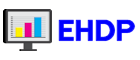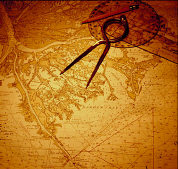Vitalnet Fill out contact form      Call 888-709-5319 Health Data Analysis SoftwareJenks Natural Breaks Explained

To help users decide which range method to use when making Vitalnet maps, (or making maps with any software), this page explains about "Natural Breaks" for setting map ranges. Jenks Natural breaks is also called "Fisher's natural breaks".

What is "Natural Breaks"? - "Natural breaks" finds the "best" way to split up the ranges. Suppose we had 30 counties, 15 counties with 0-1 values, 10 counties with 16-18 values, and 5 counties with 24-29 values. Obviously, the "best" ranges are 0-1, 16-18, 24-29. Counties with similar values should have the same color. "Natural breaks" is the only method that finds the "best" ranges.

What do we mean by "best ranges"? - It means the ranges where like areas are grouped together. Obviously, we do not give a low rate area the same color as a high rate area. Natural breaks minimizes the variation within each color, so the areas within each color are as close as possible in value to each other.

Does natural breaks make the "prettiest map"? There is no such assurance. But natural breaks makes the most epidemiologically accurate map. Making the map "pretty" is important, but that would involve such factors as right number of colors (not too few, not too many), right color palette (a standard ColorBrewer palette provided by Vitalnet), high resolution so the map does not look fuzzy, and nice legend.

How does natural breaks work? Natural breaks is complicated because many steps are involved, but does not involve any higher math. Step #1 is simple - Calculate the "sum of squared deviations for array mean" (SDAM). Assume four counties, with values: 4, 5, 9, 10. Mean = 7. SDAM = (4-7)^2 + (5-7)^2 + (9-7)^2 + (10-7)^2 = 9 + 4 + 4 + 9 = 26. Step #2 is complex - For each range combination, calculate "sum of squared deviations for class means" (SDCM_ALL), and find the smallest one. SDCM_ALL is similar to SDAM, but uses class means and deviations. Suppose we have four counties and two ranges. For [5,9,10], SDCM_ALL = (4-4)^2 + (5-8)^2 + (9-8)^2 + (10-8)^2 = 0 + 9 + 1 + 4 = 14. For [4,5][9,10], SDCM_ALL = (4-4.5)^2 + (5-4.5)^2 + (9-9.5)^2 + (10-9.5)^2 = 0.25 + 0.25 + 0.25 + 0.25 = 1. For [4,5,9], SDCM_ALL = (4-6)^2 + (5-6)^2 + (9-6)^2 + (10-10)^2 = 4 + 1 + 9 + 0 = 14. [4,5][9,10] has the smallest SDCM_ALL, so is "best ranges", minimizes variation within classes. Intuitively, it makes sense to use [4,5][9,10], and the natural breaks algorithm automatically figures this out. Step #3 is simple - As a final summary measure, calculate a "goodness of variance fit" (GVF), defined as (SDAM - SCDM) / SDAM. GVF ranges from 1 (perfect fit) to 0 (awful fit). Higher SDCM_ALL (more variation within classes) results in lower GVF. In the examples in step #2, GVF is (26 - 1) / 26 = 25 / 26 = 0.96 for the best combination, and (26 - 14) / 26 = 12 / 26 = 0.46 for the two rejected combinations, a huge difference.

What are alternatives to natural breaks? There are two widely used, but arguably inferior, alternative ways to set map ranges. Both make arbitrary cut points, and do not produce the "best ranges". However, both are at least fast to run and easy to explain. (1) Equal Count (Quantiles) - Splits the entire data span (from lowest to highest value) into equal ranges. Suppose the lowest value is 10 deaths, the highest value is 39 deaths, and there are three ranges. Range #1 would be 10-19, range #2 would be 20-29, range #3 would be 30-39. (2) Equal Interval - Sets the ranges so that an equal number of areas (eg, counties) are in each range. Suppose there are 30 counties, and three ranges. Range #1 would hold the lowest 10 counties, range #2 the middle 10 counties, range #3 the highest 10 counties.

Is it a good idea to set ranges by hand? Usually not, for two reasons. (1) Usually impractical. There are an overwhelming number of different ways to set map ranges. Suppose we had 254 counties, and 6 colors: there would be 8,301,429,675 (over eight BILLION) possible range combinations! Even with a lower number of ranges and counties, you could not possibly explore even a tiny fraction of the combinations by hand. (2) Usually inaccurate. It destroys the objective display of the data. Of the few patterns the user can test, the "prettiest" pattern will almost certainly be selected, but that has nothing to do with the correct display of the data. If the pattern resulting from natural breaks is not "pretty", it just means there is no strong geographic pattern to the data. Artificially selecting ranges to produce a "pretty" or desired pattern can easily turn into "how to lie with maps". A valid reason to manually set map ranges is to compare two maps, to use the same ranges as in an existing map.

How long does natural breaks take? - It depends on the number of areas, number of colors, and the CPU. Remember that with 254 counties and 6 colors, there are 8,301,429,675 possible range combinations. It might take the computer a little while to test so many combinations. There would be even more combinations (a lot more) with 7 colors. Vitalnet natural breaks has been designed with special programming to run as fast as possible. It runs quickly, a second or two, with a modest number of ranges or if not that many areas. But it eventually slows down with a larger number of ranges and areas. So if using natural breaks, start with a low number of ranges, and only increase the number to a large number if needed, and then be prepared for a possible wait. If needed, you can minimize the window and do other tasks.

Natural Break Examples and Benchmarking

Natural Breaks Compared with Other Methods

Useful References: (1) Dent, BD (1999) Cartography: Thematic Map Design. McGraw-Hill. (2) Slocum, TA (1999) Thematic Cartography and Visualization. Prentice-Hall.